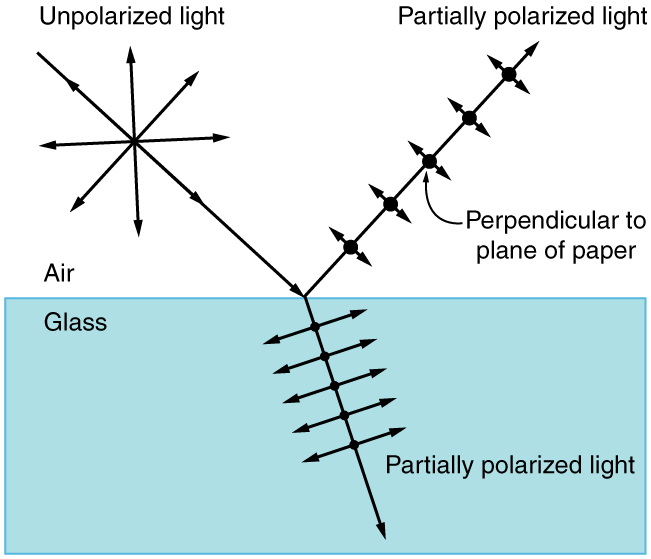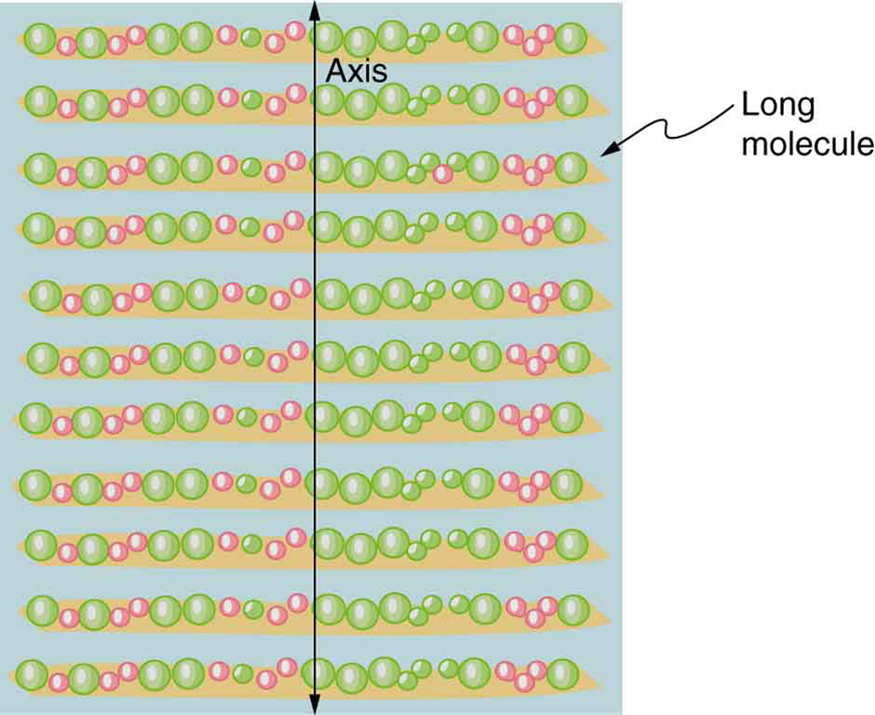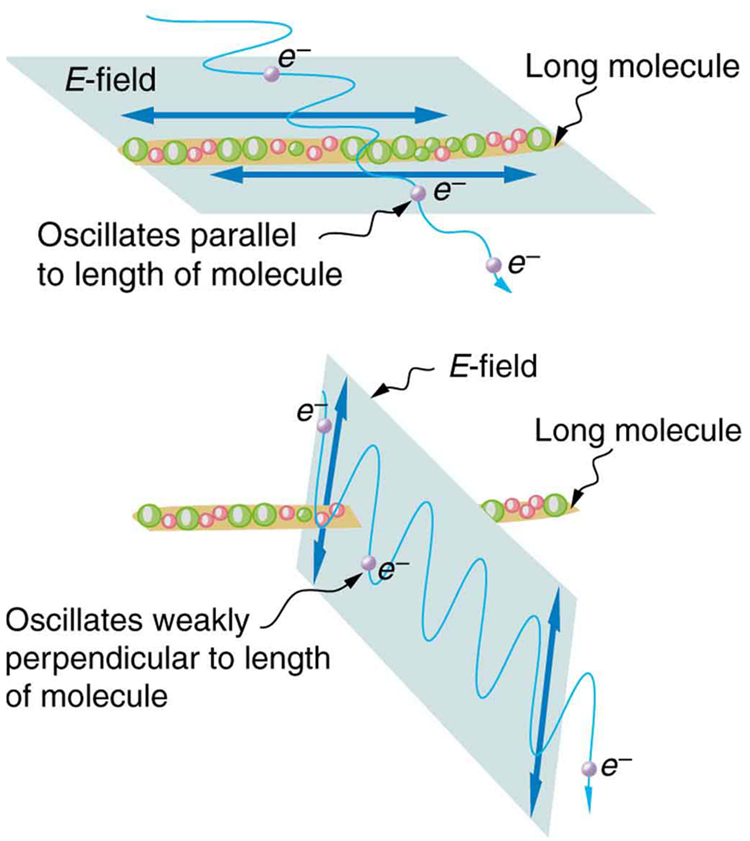# 27.8 Polarization  (Page 3/19)

 Page 3 / 19

## Polarization by reflection

By now you can probably guess that Polaroid sunglasses cut the glare in reflected light because that light is polarized. You can check this for yourself by holding Polaroid sunglasses in front of you and rotating them while looking at light reflected from water or glass. As you rotate the sunglasses, you will notice the light gets bright and dim, but not completely black. This implies the reflected light is partially polarized and cannot be completely blocked by a polarizing filter.

[link] illustrates what happens when unpolarized light is reflected from a surface. Vertically polarized light is preferentially refracted at the surface, so that the reflected light is left more horizontally polarized . The reasons for this phenomenon are beyond the scope of this text, but a convenient mnemonic for remembering this is to imagine the polarization direction to be like an arrow. Vertical polarization would be like an arrow perpendicular to the surface and would be more likely to stick and not be reflected. Horizontal polarization is like an arrow bouncing on its side and would be more likely to be reflected. Sunglasses with vertical axes would then block more reflected light than unpolarized light from other sources.Polarization by reflection. Unpolarized light has equal amounts of vertical and horizontal polarization. After interaction with a surface, the vertical components are preferentially absorbed or refracted, leaving the reflected light more horizontally polarized. This is akin to arrows striking on their sides bouncing off, whereas arrows striking on their tips go into the surface.

Since the part of the light that is not reflected is refracted, the amount of polarization depends on the indices of refraction of the media involved. It can be shown that reflected light is completely polarized at a angle of reflection ${\theta }_{\text{b}}$ , given by

$\text{tan}\phantom{\rule{0.25em}{0ex}}{\theta }_{\text{b}}=\frac{{n}_{2}}{{n}_{1}}\text{,}$

where ${n}_{1}$ is the medium in which the incident and reflected light travel and ${n}_{2}$ is the index of refraction of the medium that forms the interface that reflects the light. This equation is known as Brewster’s law    , and ${\theta }_{\text{b}}$ is known as Brewster’s angle    , named after the 19th-century Scottish physicist who discovered them.

## Things great and small: atomic explanation of polarizing filters

Polarizing filters have a polarization axis that acts as a slit. This slit passes electromagnetic waves (often visible light) that have an electric field parallel to the axis. This is accomplished with long molecules aligned perpendicular to the axis as shown in [link] .Long molecules are aligned perpendicular to the axis of a polarizing filter. The component of the electric field in an EM wave perpendicular to these molecules passes through the filter, while the component parallel to the molecules is absorbed.

[link] illustrates how the component of the electric field parallel to the long molecules is absorbed. An electromagnetic wave is composed of oscillating electric and magnetic fields. The electric field is strong compared with the magnetic field and is more effective in exerting force on charges in the molecules. The most affected charged particles are the electrons in the molecules, since electron masses are small. If the electron is forced to oscillate, it can absorb energy from the EM wave. This reduces the fields in the wave and, hence, reduces its intensity. In long molecules, electrons can more easily oscillate parallel to the molecule than in the perpendicular direction. The electrons are bound to the molecule and are more restricted in their movement perpendicular to the molecule. Thus, the electrons can absorb EM waves that have a component of their electric field parallel to the molecule. The electrons are much less responsive to electric fields perpendicular to the molecule and will allow those fields to pass. Thus the axis of the polarizing filter is perpendicular to the length of the molecule.Artist’s conception of an electron in a long molecule oscillating parallel to the molecule. The oscillation of the electron absorbs energy and reduces the intensity of the component of the EM wave that is parallel to the molecule.

#### Questions & Answers

What does mean ohms law imply
what is matter
Anything that occupies space
Kevin
Any thing that has weight and occupies space
Victoria
Anything which we can feel by any of our 5 sense organs
Suraj
Right
Roben
thanks
Suraj
what is a sulphate
Alo
Alo
the time rate of increase in velocity is called
acceleration
Emma
What is uniform velocity
Victoria
Greetings,users of that wonderful app.
how to solve pressure?
how do we calculate weight and eara eg an elefant that weight 2000kg has four fits or legs search of surface eara is 0.1m2(1metre square) incontact with the ground=10m2(g =10m2)
Cruz
P=F/A
Mira
can someone derive the formula a little bit deeper?
Bern
what is coplanar force?
what is accuracy and precision
How does a current follow?
follow?
akif
which one dc or ac current.
akif
how does a current following?
Vineeta
?
akif
AC current
Vineeta
AC current follows due to changing electric field and magnetic field.
akif
you guys are just saying follow is flow not follow please
Abubakar
ok bro thanks
akif
flows
Abubakar
but i wanted to understand him/her in his own language
akif
but I think the statement is written in English not any other language
Abubakar
my mean that in which form he/she written this,will understand better in this form, i write.
akif
ok
Abubakar
ok thanks bro. my mistake
Vineeta
u are welcome
Abubakar
what is a semiconductor
substances having lower forbidden gap between valence band and conduction band
akif
what is a conductor?
Vineeta
replace lower by higher only
akif
convert 56°c to kelvin
Abubakar
How does a current follow?
Vineeta
A semiconductor is any material whose conduction lies between that of a conductor and an insulator.
AKOWUAH
what is Atom? what is molecules? what is ions?
What is a molecule
Is a unit of a compound that has two or more atoms either of the same or different atoms
Justice
A molecule is the smallest indivisible unit of a compound, Just like the atom is the smallest indivisible unit of an element.
Rachel
what is a molecule?
Vineeta
what is a vector
A quantity that has both a magnitude AND a direction. E.g velocity, acceleration, force are all vector quantities. Hope this helps :)
deage
what is the difference between velocity and relative velocity?
Mackson
Velocity is the rate of change of displacement with time. Relative velocity on the other hand is the velocity observed by an observer with respect to a reference point.
Chuks
what do u understand by Ultraviolet catastrophe?
Rufai
A certain freely falling object, released from rest, requires 1.5seconds to travel the last 30metres before it hits the ground. (a) Find the velocity of the object when it is 30metres above the ground.
Mackson
A vector is a quantity that has both magnitude and direction
Rufus
the velocity Is 20m/s-2
Rufus
derivation of electric potential
V = Er = (kq/r^2)×r V = kq/r Where V: electric potential.
Chuks
what is the difference between simple motion and simple harmonic motion ?
syed
hi
Peace
hi
Rufus
hi
Chip
simple harmonic motion is a motion of tro and fro of simple pendulum and the likes while simple motion is a linear motion on a straight line.
Muinat
a body acceleration uniform from rest a 6m/s -2 for 8sec and decelerate uniformly to rest in the next 5sec,the magnitude of the deceleration is ?
The wording not very clear kindly
Moses
6
Leo
9.6m/s2
Jolly
the magnitude of deceleration =-9.8ms-2. first find the final velocity using the known acceleration and time. next use the calculated velocity to find the size of deceleration.
Mackson
wrong
Peace
-3.4m/s-2
Justice
Hi
Abj
Firstly, calculate final velocity of the body and then the deceleration. The final ans is,-9.6ms-2
Muinat
8x6= 48m/-2 use v=u + at 48÷5=9.6
Lawrence
can i define motion like this motion can be define as the continuous change of an object or position
Any object in motion will come to rest after a time duration. Different objects may cover equal distance in different time duration. Therefore, motion is defined as a change in position depending on time.
ChuksByByBy